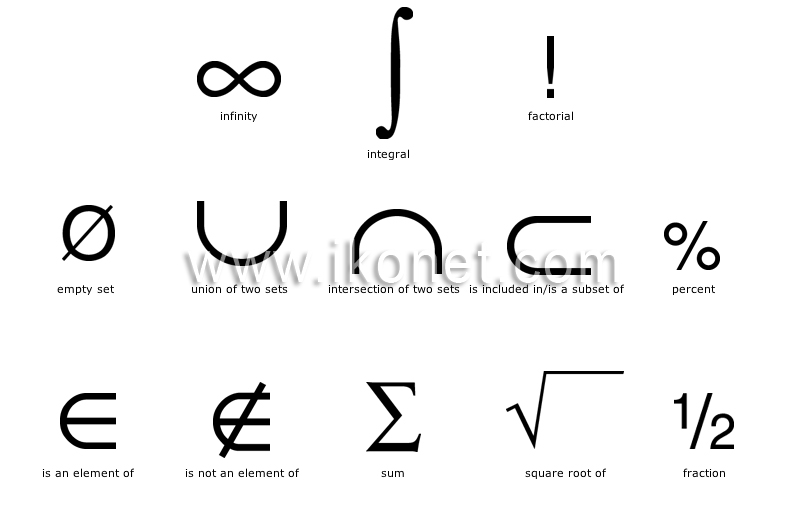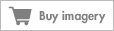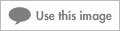Look up an image:

#### mathematicsThe science that uses deductive reasoning to study the properties of abstract entities such as numbers, space and functions and the relations between them.See mathematics in : french | spanish

#### factorialProduct of all positive whole numbers less than and equal to a given number. For example, the factorial of 4 is: 4! = 1x2x3x4 = 24.

#### integralResult of the integral calculation used especially to determine an area and to resolve a differential equation.

#### infinitySymbol denoting that a value has no upper limit.

#### fractionSign denoting that the number on the left of the slash (numerator) is one part of the number on the right of the slash (denominator).

#### square root ofSign denoting that, when a number is multiplied by itself, the result is the number that appears below the bar.

#### is not an element ofBinary sign denoting that the element on the left is not included in the set on the right.

#### is an element ofBinary sign denoting that the element on the left is included in the set on the right.

#### union of two setsBinary sign denoting that a set is composed of the sum of the elements of two sets.

#### intersection of two setsBinary sign denoting that two sets M and N have elements in common.

#### is included in/is a subset ofBinary sign denoting that a set A on the left is part of the set B on the right.

#### percentSign denoting that the number preceding it is a fraction of 100.

#### empty setSign denoting that a set contains no elements.